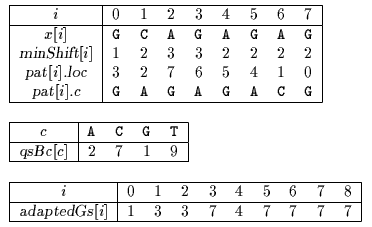Name
• String
• Substring
• Search
Edit

Sunday designed an algorithm where the pattern characters are scanned from the one which will lead to a larger shift to the one which will lead to a shorter shift. Doing so one may hope to maximize the lengths of the shifts.

The preprocessing phase of the Maximal Shift algorithm consists in sorting the pattern characters in decreasing order of their shift and then in building the Quick Search bad-character shift function (see chapter Quick Search algorithm) and a good-suffix shift function adapted to the scanning order of the pattern characters. It can be done in O(m2+) time and O(m+) space complexity.

The searching phase of the Maximal Shift algorithm has a quadratic worst case time complexity.

Main features:

• variant of the Quick Search algorithm;
• quadratic worst case time complexity;
• preprocessing phase in O(m2+) time and O(m+) space complexity.
• searching phase in O(mn) time complexity.

Example:

Preprocessing phaseTables used by Maximal Shift algorithm.

Searching phase

 G C A T C G C A G A G A G T A T A C A G T A C G 1 G C A G A G A G

 G C A T C G C A G A G A G T A T A C A G T A C G 1 G C A G A G A G

Shift by: 2 (qsBc[A])

 G C A T C G C A G A G A G T A T A C A G T A C G 1 G C A G A G A G

Shift by: 2 (qsBc[A])

 G C A T C G C A G A G A G T A T A C A G T A C G 8 7 2 1 6 5 4 3 G C A G A G A G

Shift by: 9 (qsBc[T])

 G C A T C G C A G A G A G T A T A C A G T A C G 1 G C A G A G A G

Shift by: 7 (qsBc[C])

The Maximal Shift algorithm performs 12 character comparisons on the example.

C